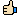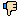Live Chat Software by Kayako
Knowledgebase
Knowledgebase:Work Flow for Assets Register Module: Posted by Syed Qutubuddin, Last modified by Kaize, Ahamed on 06 August 2019 03:46 PM Work Flow for Assets Register Module: 1)Pre-requisite: COA codes - Asset G/L Code (Balance Sheet: Assets)- Asset G/L Depreciation (Balance Sheet: Lability)- Asset G/L Depreciation (P&L: Expense)- Assets Disposal g/L (P&L: Expense) Pre-requisite: BUA and Ledger Setup - BUA ->Memo account must be entered. to any value the qty (memorandum field in LE.- Ledger Setup: select " User Assets QTY"   2) Assets Class: to create the grouping of the assets like vehicle furniture. - Assets class code. -Depreciation Account: - Balance Sheet. =liability - Profit & Loss = P&L Expense -Value 1 Depreciation ->Deprecation Setting: - Base Depreciation method: Straight Line - Base percentage (depreciation percentage): to depreciate the assets - press okay to create. 3) Assets Note: just optional to write all details about below assets record. 4) Assets Records: To create the assets, you can call the class so all the above setting will come from the class automatically. - Assets Code. - Select Assets Class (it will update above values automatically) - make it active. - Depreciation Account: B/S and P&L will come from class or otherwise can put manually. - Depreciation Period: Start period from where the period start to depreciate the assets - Base depreciation method: it could come from assets classes or you can put it manually. - Base percentage: like how much percentage, assets will depreciate each MONTH. - Base Final Value: Also, known as Salvage value: means at what value when it reaches then we need to dispose the assets. usually select 1 - FORMULA TO CALCUTE THE STRAIGHT LINE DESPRECIATION: - (Base percentage of (total value of assets-Base Final Value)/12 month (as per the period) e.g.: total Value assets= 100,000 and Dep%=10% then-> then 10% of assets value is 10,000 per annum so for each month divide 10,000 by 12 that is: 833.33. (this example if you don’t have base final value, if you have you must minus it from total assets value then calculate percentage) if you want to exclude the final value to be calculated while calculating the depreciating ->go to ledger setup and select "exclude final value from dep". Other NOTES: -base days for first peroid : 31 -base active data: 15 (as 15 days left for this month, as I receive item on 16th) Both above option is useful if you receive asset on 16th Jan, and you would like to start the depreciation from this month only then vale would be like above, 5) WORKFLOW: we will receive the assets from LE and once we receive we can see it in assets inquiry as well as in assets records the total value of assets. then we will run depreciation on this asset that we receive so it will show that assets are depreciated by the above formula. also, once your asset is totally depreciated or damaged, we can even dispose it by using the disposing functionality.In disposing: when you receive the asset, you receive by the qty (memorandum). so, when disposing you need to mention the qty and that qty will be disposed and value will be calculated accordingly. see below in details: I) Ledger Enter: Asset GL code will be debit with asset code (that is car and other, which you create under asset records). also, put asset value and qty(memorandum) also, select asset indicator: initial; Supplier will be credit as normal. II) To run the depreciation, you can create first the preset that will depreciate the assets value by department wise. (like I have 1 asset in hotel and then it is depreciated by room, hk etc so we can set the %factor for each dept.) - Creating preset: go to Asset records->click on assets posting preset-> put asset sub code, description, lookup. factor(ratio) and analysis (to select which dept. it goes) III) Once asset preset is done then, run the asset depreciation calculation: - go to the asset depreciation calculation->select assets code, depreciation period, post transaction=yes, posting period single/historic (single mean it will calculate the dep for the remaining period and post it in this period. but if you select historic then, it will calculate all the previous miss derivatization and post it in each missed months) also, put balance sheet code and P&L (same like the one we put in assets record for this asset. -> press ok and print to display the report please note if you select post transaction=NO then it will not be posted but only you can only print. once you press ok asset will be depreciated base the distribution in preset posting.you can check the report under asset inquiry. IV) dispose the partial asset or full asset: - first dispose part: go to the asset record-> and select asset code and change->asset status to assets to disposal (you can do the same in asset disposal selection) - Then go to asset part disposal-> select asset code->disposal quantity->part disposal period, P&L disposal account, post transaction=yes, suspense disposal account= same like p&L disposal account. - Once okay it will be disposed.   Report Manager:- asset book listing- Asset Record Setup- Assets Posting preset setup.(0 vote(s))HelpfulNot helpful